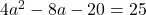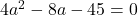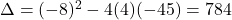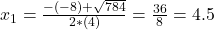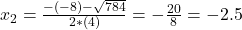## If f(x) =4×2 – 8x – 20 and g(x) = 2x + a, find the value of a so that the y-intercept of the graph of the composite function (fog)(x) is (0,

Question

If f(x) =4×2 – 8x – 20 and g(x) = 2x + a, find the value of a so that the y-intercept of the graph of the composite function (fog)(x) is (0, 25).

in progress 0
6 months 2021-08-02T15:55:20+00:00 1 Answers 4 views 0

The possible values are a = -2.5 or a = 4.5.

Step-by-step explanation:

Composite function:

The composite function of f(x) and g(x) is given by: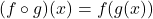In this case: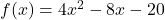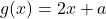So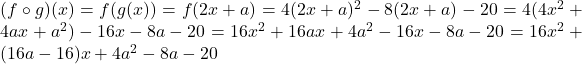Value of a so that the y-intercept of the graph of the composite function (fog)(x) is (0, 25).

This means that when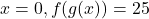. So